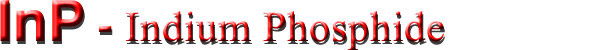## Optical properties

 Infrared refractive index 3.1 Radiative recombination coefficient 1.2·10-10 cm3/s

### Infrared refractive index

n = k1/2 = 3.075·(1+2.7·10-5T)

Long-wave TO phonon energy at 300 K hνTO = 38.1 meV
Long-wave LO phonon energy at 300 K hνLO = 42.6 meV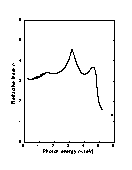Refractive index n versus photon energy. Solid curve is theoretical calculation. Points represent experimental data, 300 K (Adachi ).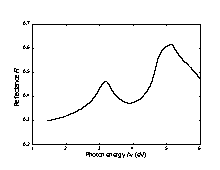Normal incidence reflectivity versus photon energy, 300 K (Aspnes and Studna ).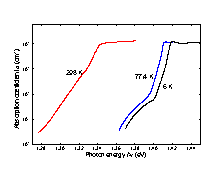Intrinsic absorption coefficient near the intrinsic absorption edge for different temperatures. n-InP. no=5·1015 cm-3 (Turner et al. ).
A ground state Rydberg energy RX1 = 5.0 meV.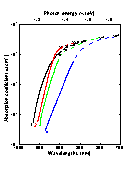Intrinsic absorption edge at 296 K at different doping levels 1. p-type sample, po = 1.1·1018 cm-3 3. n-type sample, no = 1.9·1018 cm-3 2. n-type sample, no = 7.4·1016 cm-3 4. n-type sample, no = 7·1018 cm-3 (Burkhard et al. 1982]).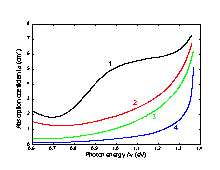Intrinsic absorption edge at 77 K for n-InP at different doping levels 1. no = 1019 cm-3; 2. no = 5·1018 cm-3; 3. no = 2·1018 cm-3; 4. no = 9.6·1016 cm-3 (Bugajski and Lewandowski ).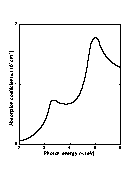The absorption coefficient versus photon energy, 300 K (Aspnes and Studna ).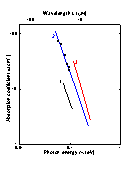Free carrier absorption versus photon energy ai different doping levels, 300 K. Electron concentration no (cm-3): 1. 4·1016; 2. 2·1017; 3. 4·1017 (Newman ).